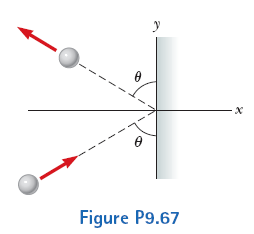# Problem: A 3.00-kg steel ball strikes a wall with a speed of 10.0 m/s at an angle of θ = 60.0° with the surface. It bounces off with the same speed and angle as shown in the figure. If the ball is in contact with the wall for 0.200 s, what is the average force exerted by the wall on the ball?

###### FREE Expert Solution
95% (419 ratings)
###### Problem Details

A 3.00-kg steel ball strikes a wall with a speed of 10.0 m/s at an angle of θ = 60.0° with the surface. It bounces off with the same speed and angle as shown in the figure. If the ball is in contact with the wall for 0.200 s, what is the average force exerted by the wall on the ball?Frequently Asked Questions

What scientific concept do you need to know in order to solve this problem?

Our tutors have indicated that to solve this problem you will need to apply the Momentum & Impulse in 2D concept. You can view video lessons to learn Momentum & Impulse in 2D. Or if you need more Momentum & Impulse in 2D practice, you can also practice Momentum & Impulse in 2D practice problems.

How long does this problem take to solve?

Our expert Physics tutor, Julia took 4 minutes and 32 seconds to solve this problem. You can follow their steps in the video explanation above.

What professor is this problem relevant for?

Based on our data, we think this problem is relevant for Professor Bennum's class at UNR.

What textbook is this problem found in?

Our data indicates that this problem or a close variation was asked in Physics for Scientists and Engineers - Serway Calc 9th Edition. You can also practice Physics for Scientists and Engineers - Serway Calc 9th Edition practice problems.